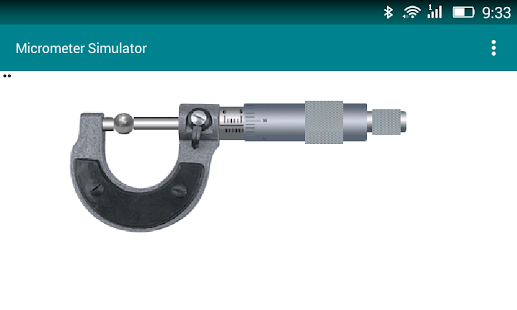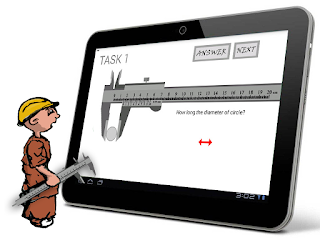## Posts

Showing posts from November, 2015

### Screw Gauge Simulator Base Android AppMicrometer Simulator is an app that used to learn about how to read Micrometer device measurement result. Teacher, student or any user else can do measurement virtually before they go to the laboratory, and do measurement with real object.  With this app they no need more time to learn how to read micrometer scale, and directly can do measurement with the real object, without go to the real laboratory.

Just drag and press the Micrometer Object to do measurement Virtually. You can change the virtual object that you want to measure with Change Object menu and try to read the measurement result on the Scale object. Have fun, and let try it!

Get it free at:
https://play.google.com/store/apps/details?id=com.priantos.micrometersimulator

You can also try the game mode of this app in link below:

### Learn How do Measure Virtually with Caliper SimulatorCaliper game is an simple game that designed to simulate how to read of Caliper measurement results. This app is one of Computational Lab of Department Physics, UB productivity app that originally purpose to increase the passion of learning about physics in Elementary Physics Lab. You can press the Caliper Image to interact with it, and learn how to measure of the different object at every task.

This game have 16 Task with different variation of measuring object, like as screw and basic object with random wide. You can also know how right your measurement result as poor, average, good or excellent by the error value at every Task. Have fun and try it free at the link below:

https://play.google.com/store/apps/details?id=com.priantos.calipergames

### Polynomial Calculator with Genetic Algorithm ImplementationPolynomial Calc is an app that designed to calculate any polynomial equation and to solve your polynomial equation with numerical approximation. This app is use numerical method, and the calculation is needed looping time and have an error value, but it can be predict many from of polynomial equation with single variable x. This is also one of Smart Calc Lib that have been developed by Computational Lab., Dept. of Physics, UB.

The main idea of this algorithm, we called as the progressive error prediction, where is the implementation of Genetic Algorithm. With that algorithm we can do the numerical calculation that have the ability to predicted many unknown variables, many inputs value and many form of equation with small error value as well as We implement on Predicted Calc App.

Just type your polynomial equations and let Polim Root find unknown x of your polynomial equation. You can get this app free at link below: